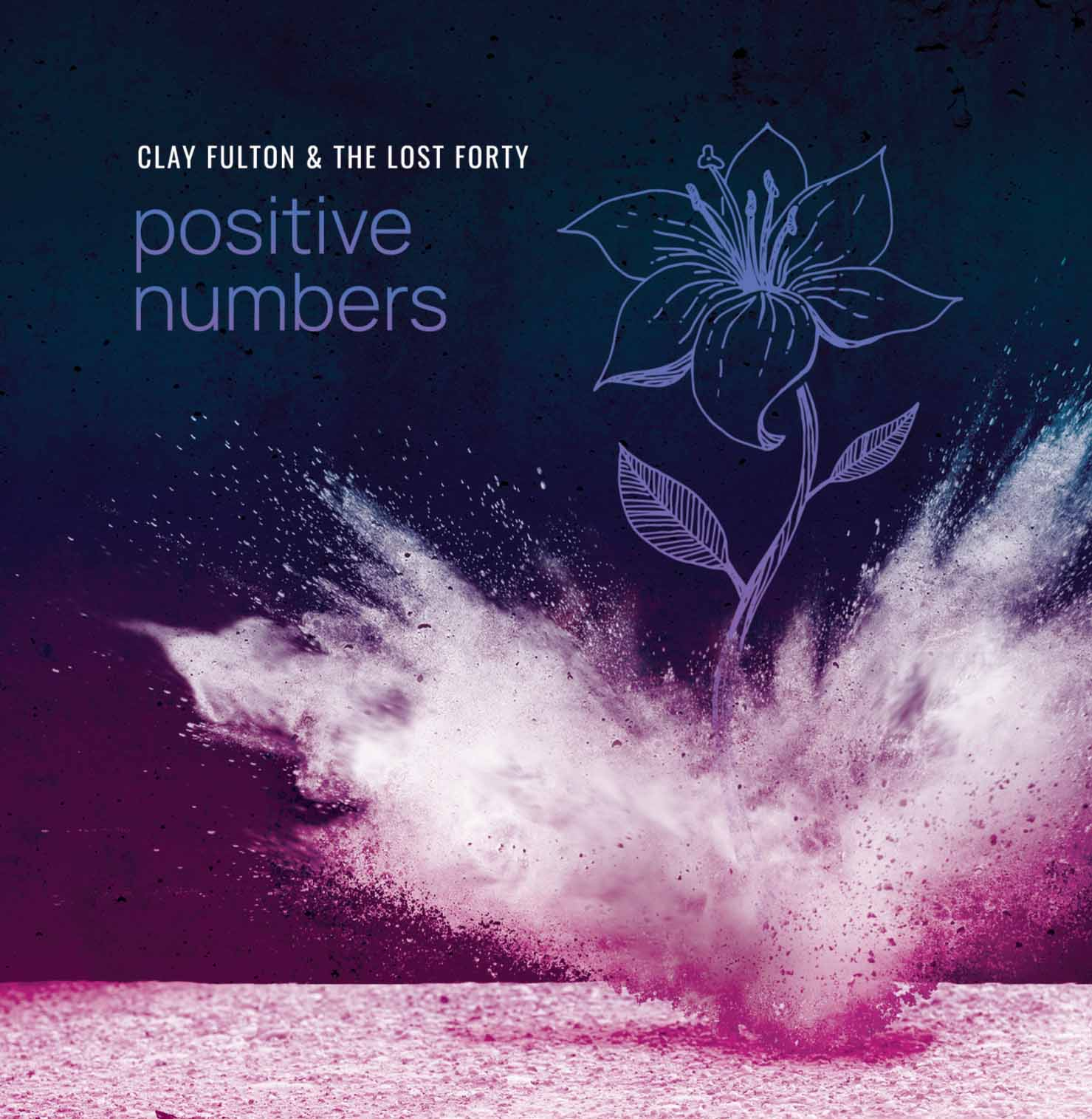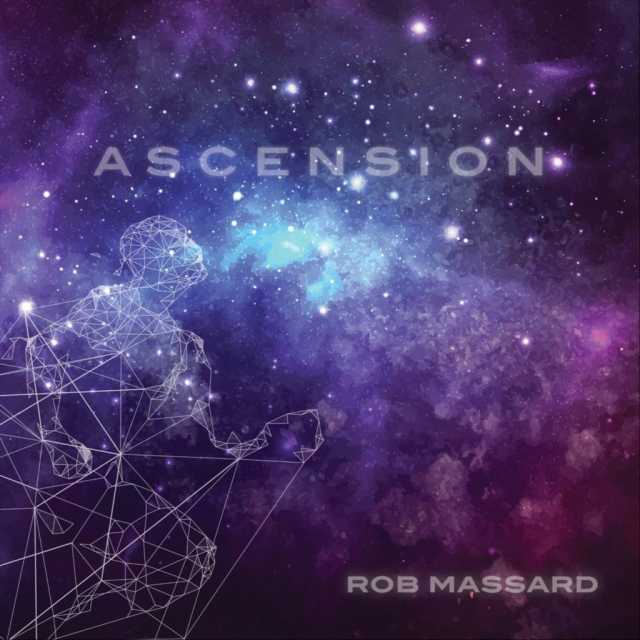Art, History, Music, Politics, and Spirituality For The Modern Alchemist – circulation in over 129 countries

# Happy New Year- Calendar of Mu – Year 18,002: A Review of the First Month (Sign of Amaterasu Ohkami)

Greetings! I would like to welcome everyone to the Art of Ninzuwu blog page. If this is your first time here, please feel free to review some of our previous articles and do not hesitate in sharing some of your thoughts and insights by posting a comment. We wish you the best in all your endeavors. Have a great day!
Today, we celebrate the Ninzuwu New Year! It is the year 18,002 in our sacred tradition. I thought it would also be a good time to explain a little bit about our calendar by examining the first month, which is labeled the Sign of Amaterasu Ohkami.

There are eight months in the Calendar of Mu, sometimes referred to as the Nyarzirian Calendar. Each month is consists of forty-five days, and represents a system of the eight hexagrams moving through the five elements. The Ninzuwu Priest/Priestess invokes the mudra/mantra formulae associated with each hexagram that rules a particular day after invoking the Armor of Amaterasu Ohkami. The chart below illustrates the daily Vasuh letters invoked and the ruling “deity” for the said hexagram:
1. March 30th, 2014 = Hahun = 14th Hexagram = Zhee-Bnhu-Aum
2. March 31st, 2014 = Shapash = 21st Hexagram =Phe-Aum-Nzu
3. April 1st, 2014 = Amaterasu = 30th Hexagram = Zhee-Lewhu
4. April 2nd, 2014 = Nuru = 35th Hexagram = Bnhu
5. April 3rd, 2014 = Yu Nin = 38th Hexagram = Phe-Aum-Shki
6. April 4th, 2014 = Kagutsuchi = 50th Hexagram = Hmu-Hmu
7. April 5th, 2014 = Fujiyama = 56th Hexagram = Bnhu-Phe
8. April 6th, 2014 = Tengu = 64th Hexagram = Zhee-Tuu-Zhee-Hmu
9. April 7th, 2014 =Entering Earth Element
10. April 8th, 2014 = Hahun = 14th Hexagram = Zhee-Bnhu-Aum
11. April 9th, 2014 = Shapash = 21st Hexagram =Phe-Aum-Nzu
12. April 10th, 2014 = Amaterasu = 30th Hexagram = Zhee-Lewhu
13. April 11th, 2014 = Nuru = 35th Hexagram = Bnhu
14. April 12th, 2014 = Yu Nin = 38th Hexagram = Phe-Aum-Shki
15. April 13th, 2014 = Kagutsuchi = 50th Hexagram = Hmu-Hmu
16. April 14th, 2014 = Fujiyama = 56th Hexagram = Bnhu-Phe
17. April 15th, 2014 = Tengu = 64th Hexagram = Zhee-Tuu-Zhee-Hmu
18. April 16th, 2014 =Entering Water Element
19. April 17th, 2014 = Hahun = 14th Hexagram = Zhee-Bnhu-Aum
20. April 18th, 2014 = Shapash = 21st Hexagram =Phe-Aum-Nzu
21. April 19th, 2014 = Amaterasu = 30th Hexagram = Zhee-Lewhu
22. April 20th, 2014 = Nuru = 35th Hexagram = Bnhu
23. April 21st, 2014 = Yu Nin = 38th Hexagram = Phe-Aum-Shki
24. April 22nd, 2014 = Kagutsuchi = 50th Hexagram = Hmu-Hmu
25. April 23rd, 2014 = Fujiyama = 56th Hexagram = Bnhu-Phe
26. April 24th, 2014 = Tengu = 64th Hexagram = Zhee-Tuu-Zhee-Hmu
27. April 25th, 2014 =Entering Fire Element
28. April 26th, 2014 = Hahun = 14th Hexagram = Zhee-Bnhu-Aum
29. April 27th, 2014 = Shapash = 21st Hexagram =Phe-Aum-Nzu
30. April 28th, 2014 = Amaterasu = 30th Hexagram = Zhee-Lewhu
31. April 29th, 2014 = Nuru = 35th Hexagram = Bnhu
32. April 30th, 2014 = Yu Nin = 38th Hexagram = Phe-Aum-Shki
33. May 1st, 2014 = Kagutsuchi = 50th Hexagram = Hmu-Hmu
34. May 2nd, 2014 = Fujiyama = 56th Hexagram = Bnhu-Phe
35. May 3rd, 2014 = Tengu = 64th Hexagram = Zhee-Tuu-Zhee-Hmu
36. May 4th, 2014 =Entering Air Element
37. May 5th, 2014 = Hahun = 14th Hexagram = Zhee-Bnhu-Aum
38. May 6th, 2014 = Shapash = 21st Hexagram =Phe-Aum-Nzu
39. May 7th, 2014 = Amaterasu = 30th Hexagram = Zhee-Lewhu
40. May 8th, 2014 = Nuru = 35th Hexagram = Bnhu
41. May 9th, 2014 = Yu Nin = 38th Hexagram = Phe-Aum-Shki
42. May 10th, 2014 = Kagutsuchi = 50th Hexagram = Hmu-Hmu
43. May 11th, 2014 = Fujiyama = 56th Hexagram = Bnhu-Phe
44. May 12th, 2014 = Tengu = 64th Hexagram = Zhee-Tuu-Zhee-Hmu
45. May 13th, 2014 =Entering Void Element

#### You may have missed#### October by Mae Simpson#### Night Owl by Adrian John Szozda